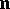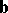# Darboux vector

(diff) ← Older revision | Latest revision (diff) | Newer revision → (diff)

The vectorof the instantaneous axis of rotation around which the natural trihedral of a curveis rotating during the uniform movement of a pointalong the curve. The Darboux vector lies in the rectifying plane of the curveand is expressed in terms of the principal normaland the tangentofby the formulawhereandare the curvature and the torsion ofandis the angle between the Darboux vector and the tangent to. The Frénet formulas may be written with the aid of the Darboux vector as follows:whereis the binormal of.

G. Darboux  was the first to point out the geometric significance of the Darboux vector for the natural trihedral of a space curve.

How to Cite This Entry:
Darboux vector. Encyclopedia of Mathematics. URL: http://encyclopediaofmath.org/index.php?title=Darboux_vector&oldid=14668
This article was adapted from an original article by E.V. Shikin (originator), which appeared in Encyclopedia of Mathematics - ISBN 1402006098. See original article